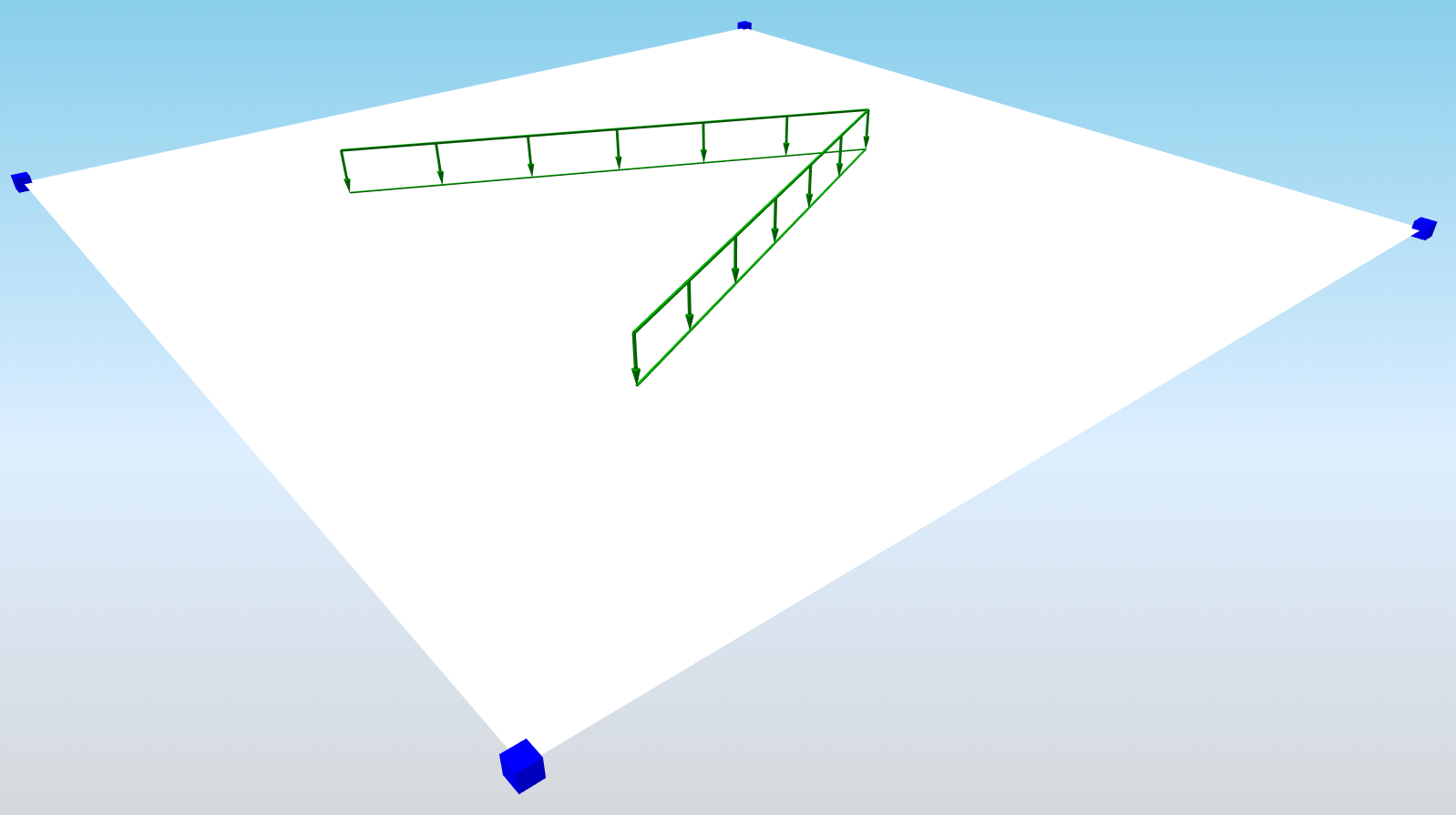# StructuralCurveActionFree¶

Free line force

The Free line load is related to slabs. The load is not defined by the entity it acts on, but by a specific load border. Free loads are defined by means of “loading entities” that may overlap or affect one or more slabs.## Specification in the excel¶

Data type

Example / enum definition

Required

Description

Name

String

LF5

yes

Human readable unique name of the force

Type

String

Standard

no

This property defines what the load is caused by, E.g. Standard, Wind, Snow, Self weight, Hoar Frost, Predefined, Plane Load, Water Pond, Water Pressure, Soil Pressure, Generated Water, Generated Soil

Distribution

Enum

Uniform

Trapez

yes

The load may be either constant along its length or linearly variable (trapezoidal).

Direction

Enum

X

Y

Z

Vector

yes

Specifies the base direction of the load

X, Y, Z - action will be applied in one of these directions

Vector - size and direction calculated from vector

Value 1 [kN/m]

Double

-150

yes, if Direction = X,Y or Z

Specifies the first size of the load in kiloNewtons per meter

Value 2 [kN/m]

Double

-180

yes, if Direction = X,Y or Z

and Distribution = Trapez

Specifies the second size of the load in kiloNewtons per meter

Vector 1(X;Y;Z) [kN/m]

String

(10;10;0)

yes, if Direction = Vector

Specifies the first size of the load in, direction by vector

Note: Vector1 and Vector2 needs to be in same direction

Vector 2(X;Y;Z) [kN/m]

String

(20;20;0)

yes, if Direction = Vector

and Distribution = Trapez

Specifies the second size of the load in, direction by vector

Note: Vector1 and Vector2 needs to be in same direction

String

LC1

yes

The name of the load case to which the force belongs

Coordinate X [m]

String

0.000; 2.050; 4.850; -2.000

yes

The list of X coordinates of the nodes which define the geometry of the line free load. Each coordinate is separated by semicolon and space.

Coordinate Y [m]

String

0.500; 1.050; 2.650; -1.500

yes

The list of Y coordinates of the nodes which define the geometry of the line free load. Each coordinate is separated by semicolon and space.

Coordinate Z [m]

String

0.000; 0.000; 0.000; 0.000

yes

The list of Z coordinates of the nodes which define the geometry of the line free load. Each coordinate is separated by semicolon and space.

Segments

String

Line; Circular Arc; Bezier; Parabolic arc; Spline

yes

List of the shape of each segment which is part of the curve, separated by semicolon if there are multiple

Coordinate system

Enum

Global

Local

yes

Defines co-ordinate system of the member in which the load is applied

Location

Enum

Length

Projection

yes

Specifies whether the load is “put directly on an inclined 1D member” or whether the “projection on plan” is defined.

Id

String

39f238a5-01d0-45cf-a2eb-958170fd4f39

no

Unique attribute designation